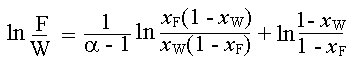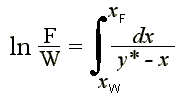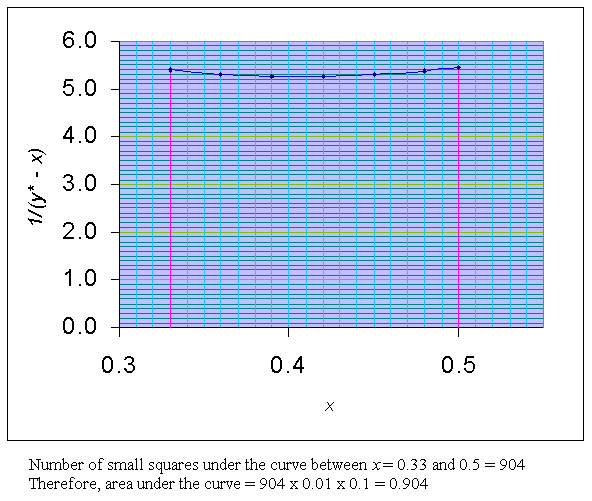### Differential Distillation

100 moles of Benzene (A) and Toluene mixture containing 50% (mole) of Benzene is subjected to a differential distillation at atmospheric pressure till the composition of benzene in the residue is 33%. Calculate the total moles of the mixture distilled. Average relative volatility may be assumed as 2.16

Calculations:

For system of constant relative volatility, the following equation is obtained after the simplification of Rayleigh's equation for differential distillation:In the above equation, F is feed; W is residue; xF is composition of feed; xW is composition of residue; and a is relative volatility.

Given: xF = 0.5; xW = 0.33; F = 100; a = 2.16

Substituting these in the above equation,

ln (100/W) = (1/1.16) x ln {[(0.5(1 - 0.33)]/[(0.33(1 - 0.5)]} + ln [(1 - 0.33)/(1 - 0.5)]

ln (100/W) = 0.862 x ln (2.03) + ln (1.34)

ln (100/W) = 0.862 x 0.708 + 0.2927

ln (100/W) = 0.903

100/W = e0.903

100/W = 2.467

W = 100/2.467 = 40.54 mole

Moles of mixture distilled = F - W = 100 - 40.54 = 59.46

The above can also be obtained by using the general form of Rayleigh's equation and by graphical method. This is given below.

The equilibrium curve relationship is given by

y* = ax/(1 + x(a - 1)) = 2.16x/(1 + 1.16x) à 1

For differential distillation, the following Rayleigh's equation is applicable:à 2

By using Equn.1 the following equilibrium data is obtained: (for the region of x = 0.33 to 0.5)

 x y* 0.33 0.515 0.36 0.549 0.39 0.580 0.42 0.610 0.45 0.639 0.48 0.666 0.5 0.684

For the above data the following table of x Vs.1/(y* - x) is obtained:

 x 1/(y* - x) 0.33 5.392 0.36 5.304 0.39 5.263 0.42 5.263 0.45 5.301 0.48 5.377 0.5 5.448

Using the above data a graph of x Vs.1/(y* - x) is drawn between the limits of x = 0.33 to 0.5.Area under the curve between the limits of x = 0.33 to 0.5, is = 0.904

i.e.,

ln (F/W) = 0.904

e0.904 = F/W

2.4695 = F/W

Given F = 100 moles. Therefore,

W = 100 / 2.4695 = 40.5 mole.

Therefore, moles of mixture distilled = F - W = 100 - 40.5 = 59.5